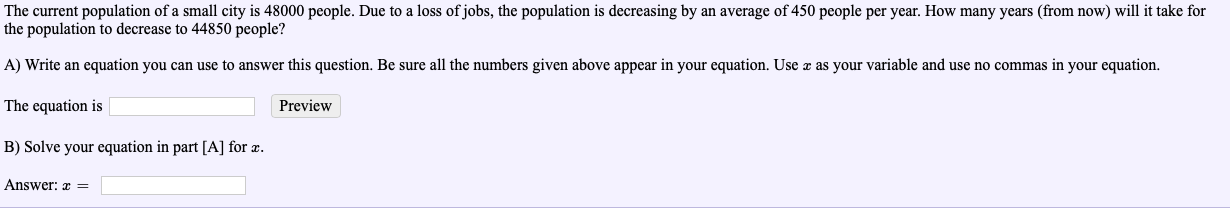# The current population of a small city is 48000 people. Due to a loss of jobs, the population is decreasing by an average of 450 people per year. How many years (from now) will it take forthe population to decrease to 44850 people?A) Write an equation you can use to answer this question. Be sure all the numbers given above appear in your equation. Use z as your variable and use no commas in your equation.The equation isB) Solve your equation in part [A] for z.PreviewAnswer: E

Questionhelp_outlineImage TranscriptioncloseThe current population of a small city is 48000 people. Due to a loss of jobs, the population is decreasing by an average of 450 people per year. How many years (from now) will it take for the population to decrease to 44850 people? A) Write an equation you can use to answer this question. Be sure all the numbers given above appear in your equation. Use z as your variable and use no commas in your equation. The equation is B) Solve your equation in part [A] for z. Preview Answer: E fullscreen
check_circleExpert Solution
Step 1

Convert the given word problem to an equation in x (the number of years) and solve for x.

Step 2

The average population decrease is 450. So, the total population decrease in x years is 450x

Step 3

Therefore, the population after x years is 4...

### Want to see the full answer?

See Solution

#### Want to see this answer and more?

Solutions are written by subject experts who are available 24/7. Questions are typically answered within 1 hour*

See Solution
*Response times may vary by subject and question
Tagged in

### Algebra